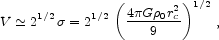2. CORE PARAMETERS OF DM HALOS

Consider first the rotation curve of an isothermal sphere in the ideal case where we can measure a massless disk embedded in it. Then at r << rc,(1)

and at r >> rc,(2)

where G is the gravitational constant. If we observe only the Vr part of the rotation curve, we can measure0 but not rc or. Because of this,0 is often the only halo parameter that we can measure in low-luminosity galaxies. In contrast, if the measurements reach far enough into the V = constant part of the rotation curve, then all three parameters can be measured. Dwarf Sc - Im galaxies come closest to the above ideal, because visible matter contributes only a small fraction of the total mass.

More generally, visible matter dominates the central part of the rotation curve, and a multicomponent mass model is required. The rotation curve of the visible matter is calculated from the brightness distribution assuming that the mass-to-light ratio M / L of each component is constant with radius. Values of M / L are adjusted to fit as much of the inner rotation curve as desired. HI gas is taken into account separately. Molecular gas is assumed to follow the light distribution, so it is included in M / L. Then, given the total rotation curve Vvis of the visible matter, the halo rotation curve is VDM(r) = (V2 - Vvis2)1/2. A model such as an isothermal is then fitted to VDM to derive the halo asymptotic velocity V= 21/2, rc, and0. Rotation curve decompositions have now been published for ~ 100 galaxies. Of these, 55 survive our selection cuts (Section 2.3).